# SAT Identifying Sentence Errors: Test 2

NOTE: The new 2016 SAT no longer contains these types of questions. Click here for our updated SAT Writing & Language practice questions.

Directions: These SAT practice questions will test your ability to recognize grammar and usage errors. Each sentence contains either a single error or no error. None of the sentences contain more than one error. If there is an error, it will be underlined and lettered. Select the underlined portion that needs to be changed in order to make the sentence correct. If the sentence is already correct, then select choice E.

Congratulations - you have completed . You scored %%SCORE%% out of %%TOTAL%%. Your performance has been rated as %%RATING%%
 Question 1A A B B C C D D E E
Question 1 Explanation:
The correct answer is (B). Use “between” when referring to two items; “among” is correct for three or more. Logic tells us that “all of the member of the faculty” would be more than two people, so (B) should be “among.” This is a “diction” error. (A) agrees with its singular subject, “No one.” (C) is correct idiomatic usage. (D) properly uses the superlative (-est) adjective form to refer to “all of the members of the faculty.”
 Question 2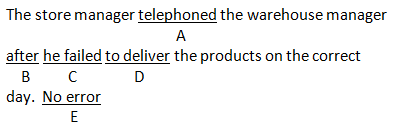A A B B C C D D E E
Question 2 Explanation:
The correct answer is (C). A pronoun that could logically refer to more than one nouns will be an Ambiguity error on the SAT. The pronoun “he” here could refer to either of the two managers; the error is in (C). (A) uses an appropriate verb tense in context. (B) properly sequences the events in the sentence. (D) is idiomatically correct usage in context.
 Question 3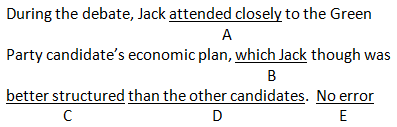A A B B C C D D E E
Question 3 Explanation:
The correct answer is (D). Make sure comparisons are structured to compare logical items. Here, one candidate’s economic plan is compared to other candidates, rather than their plans. (D) should read “than those of the other candidates.” (A) properly uses an adverb to modify a verb. (B) uses the appropriate pronoun to refer to “plan.” (C) is idiomatically correct usage.
 Question 4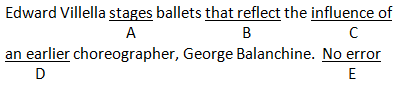A A B B C C D D E E
Question 4 Explanation:
The correct answer is (E). Between 5-8 sentences in your SAT Writing sections will contain no error. The verbs in (A) and (B) agree with their subjects, “Edward Villella” and “ballets,” respectively. (C) and (D) are idiomatically correct in context. This sentence is correct as written.
 Question 5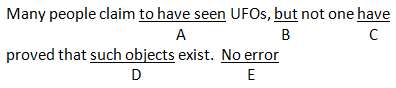A A B B C C D D E E
Question 5 Explanation:
The correct answer is (C). Most SAT sentences will contain multiple nouns and verbs; make sure each verb agrees with the appropriate noun. The plural verb “have” in (C) does not agree with its singular subject “one”; the verb should be “has.” (A) and (D) are idiomatically correct usage. (B) properly reflects the contrast between the ideas in the two clauses.
 Question 6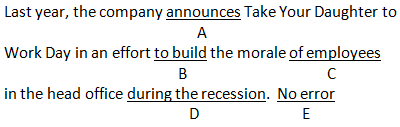A A B B C C D D E E
Question 6 Explanation:
The correct answer is (A). Make sure that verb tenses properly sequence the events discussed in a sentence. Since the company’s announcement took place “last year,” the present tense verb in (A) is incorrect; it should read “announced.” (B), (C), and (D) are all idiomatically correct usage in context.
 Question 7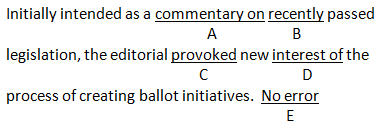A A B B C C D D E E
Question 7 Explanation:
The correct answer is (D). Many SAT idioms will be correctly structured but incorrect in the context in which they are used. Although you could provoke the “interest of” a person or group, you provoke “interest in” a process; the error is in (D). (A) is correct idiomatic usage. (B) properly uses an adverb to modify a verb form. (C) uses a consistent verb tense.
 Question 8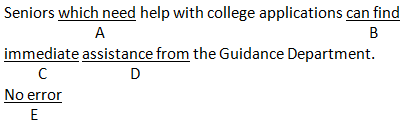A A B B C C D D E E
Question 8 Explanation:
The correct answer is (A). When referring to people, use “who” or “whom.” (A) contains the error; since the pronoun here refer to “Seniors,” “which” should be “who.” (B) is an appropriate verb tense in context. (C) properly uses an adjective to modify a noun. (D) is correct idiomatic usage.
 Question 9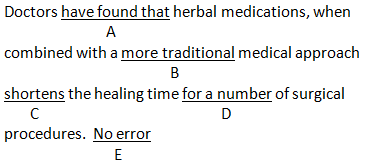A A B B C C D D E E
Question 9 Explanation:
The correct answer is (C). The SAT will sometimes use long intervening phrases to separate a subject and a verb that do not agree. Here, the singular verb “shortens” does not agree with its plural subject “medications”; (C) should read “shorten.” (A) agrees with its plural subject, “Doctors.” (B) and (D) are idiomatically correct in context.
 Question 10A A B B C C D D E E
Question 10 Explanation:
The correct answer is (D). In a comparison, make sure the objects being compared are logical. This sentence compared “The complex formulas of Algebra II” with “Algebra I.” A correct comparison would either be between the two courses or their formulas and problems; the error is in (D). (A) and (C) are correct idiomatic usage. (B) is an appropriate verb tense in context.
Once you are finished, click the button below. Any items you have not completed will be marked incorrect.
There are 10 questions to complete.
 ← List →